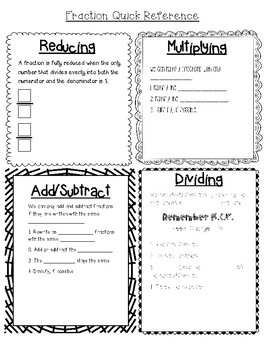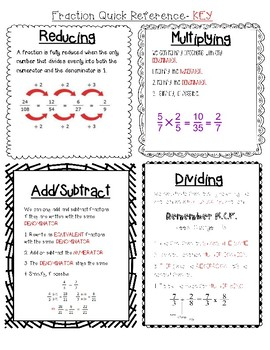# Fraction Operation IMN Reference SheetSubject
Resource Type
File Type

PDF

(450 KB|3 pages)
Product Rating
Standards
• Product Description
• StandardsNEW
A quick note taking aid for fraction operations. Great for a IMN or as a hand out to quickly review fraction operations.
Interpret the product (𝘢/𝘣) × 𝘲 as a parts of a partition of 𝘲 into 𝘣 equal parts; equivalently, as the result of a sequence of operations 𝘢 × 𝘲 ÷ 𝘣. For example, use a visual fraction model to show (2/3) × 4 = 8/3, and create a story context for this equation. Do the same with (2/3) × (4/5) = 8/15. (In general, (𝘢/𝘣) × (𝘤/𝘥) = 𝘢𝘤/𝘣𝘥.)
Interpret a fraction as division of the numerator by the denominator (𝘢/𝘣 = 𝘢 ÷ 𝘣). Solve word problems involving division of whole numbers leading to answers in the form of fractions or mixed numbers, e.g., by using visual fraction models or equations to represent the problem. For example, interpret 3/4 as the result of dividing 3 by 4, noting that 3/4 multiplied by 4 equals 3, and that when 3 wholes are shared equally among 4 people each person has a share of size 3/4. If 9 people want to share a 50-pound sack of rice equally by weight, how many pounds of rice should each person get? Between what two whole numbers does your answer lie?
Add and subtract mixed numbers with like denominators, e.g., by replacing each mixed number with an equivalent fraction, and/or by using properties of operations and the relationship between addition and subtraction.
Understand addition and subtraction of fractions as joining and separating parts referring to the same whole.
Recognize and generate simple equivalent fractions, (e.g., 1/2 = 2/4, 4/6 = 2/3). Explain why the fractions are equivalent, e.g., by using a visual fraction model.
Total Pages
3 pages
Included
Teaching Duration
N/A
Report this Resource to TpT
Reported resources will be reviewed by our team. Report this resource to let us know if this resource violates TpT’s content guidelines.